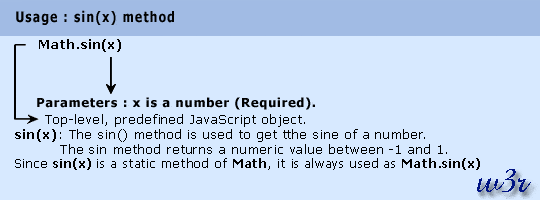# JavaScript sin() Method

## Description

The sin() method of math object is used to get the sine of a number. The sin method returns a numeric value between -1 and 1, which represents the sine of the argument.

Version

Implemented in JavaScript 1.0

Syntax

`sin(x)`

Parameter

x : Refers a number.Example:

In the following web document, sin() method returns the sine of various numbers.

``````<!DOCTYPE html PUBLIC "-//W3C//DTD XHTML 1.0 Transitional//EN"
"http://www.w3.org/TR/xhtml1/DTD/xhtml1-transitional.dtd">
<html xmlns="http://www.w3.org/1999/xhtml" xml:lang="en" lang="en">
<meta http-equiv="content-type" content="text/html; charset=iso-8859-1" />
<title>JavaScript Math object - sin() method example</title>
<body>
<h1 style="color: red">JavaScript Math object : sin() method</h1>
<hr />
<script type="text/javascript">
//This is done to make the following JavaScript code compatible to XHTML. <![CDATA[
document.write(Math.sin(3) + "<br />");
document.write(Math.sin(-3) + "<br />");
document.write(Math.sin(0) + "<br />");
document.write(Math.sin(Math.PI) + "<br />");
document.write(Math.sin(Math.PI/2) + "<br />");
document.write(Math.sin(Math.PI/3));
//]]>
</script>
</body>
</html>
```
```

View the example in the browser

Supported Browser

 Internet Explorer 7 Firefox 3.6 Google Chrome 7 Safari 5.0.1 Opera 10 Yes Yes Yes Yes Yes